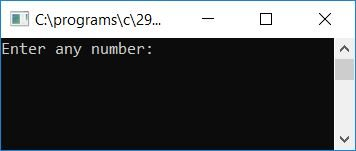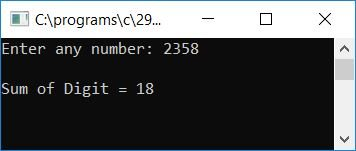# C program to find and print the sum of all the digits of any number

Here we will learn about how to create a program in C that will ask the user to enter any number (at run-time) as input and then calculate and print the sum of all the digits present in that given number. Here is the program:

```#include<stdio.h>
#include<conio.h>
int main()
{
int num, sum=0, rem;
printf("Enter any number: ");
scanf("%d", &num);
while(num>0)
{
rem = num%10;
sum = sum + rem;
num = num/10;
}
printf("\nSum of Digit = %d", sum);
getch();
return 0;
}```

As the program was written under the Code::Blocks IDE, therefore, after a successful build and run, here is the output:Supply any number, say 2358, and press the ENTER key to see the sum of all the digits as the output or result. Here is the second snapshot of the sample run:Here is a list of some of the main steps used in the above program:

• Receive any number from the user at run-time as input.
• Create a while loop that runs until the number is greater than 0.
• Inside the while loop, find the digit of the number by applying the remainder or modulus (%) operator, that is, use num%10, which gives the last digit of the number and will be initialized to the variable rem. Initialize sum+rem to the sum variable. Never forget to initialize 0 to sum at the start of the program. And then divide the number by 10 and continue to check and do the same operation.
• Let's suppose that the user has supplied 247 as input.
• If the variable num has a value greater than 0, the program flow enters the while loop, and num%10 or 247%10 or 7 is initialized to rem and sum+rem or 0+7  or 7 will be initialized to the variable sum. And finally, num/10, 247/10, or 24 will be initialized to num.
• At the second run of the while loop, num holds 24, which is again greater than 0, then the program flow again goes inside the while loop and does the same task in the same way as it did at the first run of the while loop.
• At last, after coming out of the while loop, the variable sum holds the value that is equal to the sum of all the digits of the number given by the user at the start of the program.

C Quiz

« Previous Program Next Program »